# Sulzer White paper 4 / 2018

## Predict distillation  tray efficiency

October 10, 2018

Have you ever wondered how Sulzer is able to design distillation columns for all the various applications so the columns work properly? It is indeed a challenging task for our engineers and consists of two major steps.

First, engineers need to determine the diameter of the column so the expected amount of product can be processed. This is done with the help of SULCOL, a Sulzer proprietary hydraulic rating program.

Second, the engineers must ensure that the column has adequate separation power to achieve the product purities required. For trays, a value called section efficiency must be applied, which is, in many cases, based on experience and is part of Sulzer’s expertise.

In theory, the section efficiency used in step two can be determined based on fundamental mass transfer considerations. However, this method is complicated, and models do not yet achieve the required accuracy. That is why engineers often make use of simple empirical models to estimate the efficiency. The O’Connell method is probably the most commonly used correlation though until now, there had been no theoretical justification as to why it works in many cases.

During my sabbatical in 2016 at Clarkson University (USA), I had the chance to work scientifically on the topic, with one of the most widely recognized experts in the field of multicomponent mass transfer, Professor Ross Taylor. Several conference proceedings and papers resulted from my collaboration with him.

With the following whitepaper, we want to shed light on why the empirical method of O'Connell from 1946 works—revealing the so far unrecognized ties to a more fundamental model. With this new insight, we have been able to modify the correlation to improve its predictive power.

#### Author

Markus Duss, Sulzer Chemtech Ltd, Winterthur, Switzerland

#### Co-Author

RosTaylor, s Clarkson University, Potsdam, New York, United States

#### Presentation

Adapted from an article published in CEP Magazine, July 2018.

https://www.aiche.org/resources/publications/cep/2018/july/predict-distillation-tray-efficiency

#### Abstract

Process engineers predominately use software based on the concept of theoretical or equilibrium stages to design distillation towers. (A theoretical stage is a hypothetical box assuming that the outlet streams are in thermodynamic equilibrium.) Such simulations allow the determination of the heat and mass balance for each theoretical stage and how many such stages are required to achieve specified product purities.

Real distillation columns are equipped either with trays or with packing. Hydraulic rating programs allow estimation of the column diameter so that the desired throughput (capacity) can be achieved with the selected tower internals. The height of the column depends on the number of theoretical trays found using a simulation and on the efficiency of the installed mass transfer device. For columns equipped with trays, the so-called section efficiency (ηsection) is require to translate the number of equilibrium stages (nth) to a number of actual trays (nactual)which is defined as:

Whenever possible process engineers rely on experience to determine section efficiencies. Though section efficiencies can be calculated based on fundamental mass transfer theory, this requires considerable additional information, some of which is not always readily available. Rigorous models have rarely been applied due to the high effort for their implementation and more importantly, because they do not achieve the accuracy required for industrial use.

In 1946 H.E. O’Connell published a graph that showed that observed section efficiencies correlate well using only two variables, namely the liquid viscosity, μL (in cP) and the relative volatility, α (dimensionless). The following correlation is well known as the “O’Connell correlation”:

O’Connell’s approach using liquid viscosity and relative volatility had no theoretical justification. In particular, the use of α, which is a thermodynamic quantity, appears somewhat unexpectedly; theoretical considerations would suggest using the stripping factor, λ. (The stripping factor is the ratio of the slope of the equilibrium line and the slope of the operating line.) However, due to its simplicity and success in predicting the section efficiency, the O’Connell correlation became probably the most widely applied method. O’Connell’s method achieves conservative values in most cases, so that columns designed on his approach often achieve the required separation duty.

This paper will derive a simplified theoretical model, based on the traditional approach, i.e. using the number of transfer units, point efficiency and tray efficiency to retrieve the section efficiency. One simplification is the assumption that the section efficiency is independent of the tray hydraulics, which is confirmed by experiments. The relative volatility that appears in O’Connell’s correlation is, here, replaced by the stripping factor, which appears naturally in the underlying theory.

This simplified theoretical model only requires regression of the number of transfer units, using the liquid viscosity as the only variable. Two parameters were fitted to minimize the deviation with O’Connell’s correlation. The outcome of the regression showed that both approaches achieve similar section efficiencies. This was an unexpected outcome. The paper will explain in more details that an apparent symmetry in the theoretical model is the key to understanding the similar outcome from the two different approaches: the section efficiency is almost identical whether the stripping factor or the reciprocal value is used. This symmetry exists only when the number of transfer unit is the same for the liquid and vapour phases. The reason why O’Connell’s method works as well as it does is that a mimics the stripping factor in the stripping section of the column, and mimics the reciprocal of the stripping factor in the rectifying section.

Based on the insight from our analysis, a modification of O’Connell’s method is proposed, using the stripping factor instead of the relative volatility with a modified exponent:

With: if λ > 1 : σ = λ and if λ < 1 : σ = 1/λ

With this modification, the simplified theoretical model and the modified O’Connell correlation can achieve close to identical results. The modified O’Connell method proposed here also achieves a better agreement with experimental data and allows the correlation to be used in absorption and stripping columns as well as in distillation (something not true of the original O’Connell method).

Finding the reason for the success of O’Connell’s correlation and its connections to traditional theoretical efficienciesapplicability. justifies its use in distillation applications while also revealing its limitations. This leads to an improved correlation that is as simple as the original but which is more accurate and has wider applicability.

#### Nomenclature

c1          = proportionality constant

G          = gas throughput per cross-sectional bubbling area, kmol/m2-s

kG         = gas-side mass-transfer coefficient, kmol/m2-s

kL          = liquid-side mass-transfer coefficient, kmol/m2-s

KOG       = overall gas-side mass-transfer coefficient, kmol/m2-s

L           = liquid throughput per cross-sectional bubbling area, kmol/m2-s

LPR      = liquid-phase resistance

m          = slope of the equilibrium line

NG         = number of transfer units in gas phase

NL         = number of transfer units in liquid phase

NOG       = overall number of transfer units in gas phase

nth         = number of theoretical stages

ntrays     = number of actual trays

x1          = exponent to be applied to the liquid viscosity

##### Greek Letters

α       = relative volatility

ηpoint    = Murphree vapor point efficiency

ηtray     = Murphree vapor tray efficiency

ηsection = section efficiency or overall efficiency

μ         = viscosity, cP

λ          = stripping factor = mG/L

σ         = either stripping or absorption factor used in Eq. 15

##### Subscripts

= gas or vapor

L         = liquid

#### 1. Introduction

Distillation — the most important unit operation for separating liquid mixtures — is carried out in columns equipped with either packing or trays.

In a conventional tray column, vapor rises vertically up through the column while the liquid flows downward and across the trays in alternate directions on successive trays (Fig. 1).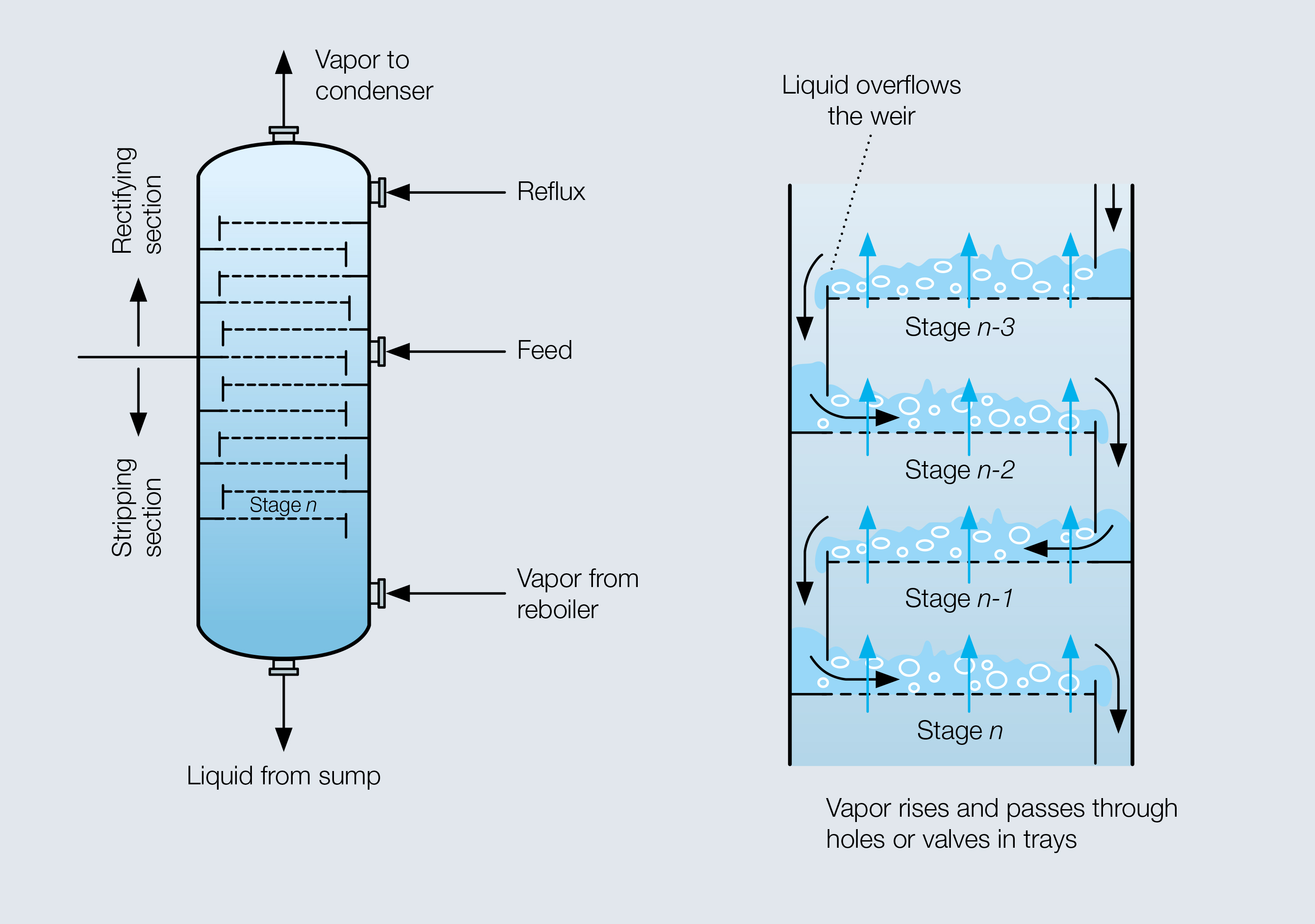Fig. 1 Distillation takes place in a column with cross-flow trays over which liquid flows in alternate directions on successive trays. The topmost tray has the highest concentration of the more-volatile species and the lowest tray has the highest concentration of the less-volatile species.
Column simulation and design software calculates the number of theoretical stages and the section efficiency is used as an efficiency factor to translate theoretical stages to actual trays. The efficiency of a particular section of a column (ηsection) is the number of theoretical stages (nth) found in a section of a column divided by the number of actual trays (ntrays):

O’Connell published a graph showing section efficiencies for bubble cap trays, which can be represented by :

where μL is the liquid viscosity in cP and α is the relative volatility.

The relative volatility and the liquid viscosity serve as the only input variables in calculating conventional distillation tray efficiencies with the empirical correlation developed by O’Connell . This simple method has survived all attempts at being replaced by more rigorous models, despite lacking a sound theoretical explanation for its success. This article presents a simple, but fundamentally sound, theoretical approach that achieves results equivalent to O’Connell’s correlation using the same number of variables — revealing the hitherto unrecognized relationship between O’Connell’s empirical correlation and other theoretical models. In addition, the article introduces a modification to O’Connell’s correlation that produces a close-to-perfect match between the updated correlation and the derived theoretical model.

#### 2. The traditional Murphree efficiency approach to tray design

The section efficiency is calculated based on fundamental mass-transfer considerations, including the approach to equilibrium both locally on the tray (point efficiency) and on the tray as a whole (tray efficiency). The conventional method of designing trays  employs Murphree’s definition of efficiency to quantify the approach to equilibrium. The overall number of transfer units for the gas phase (NOG) is :

where NG and NL represent the number of transfer units in the gas and liquid phases (a measure to quantify mass transfer), respectively, and λ is the stripping factor calculated as λ = mG/L, where m is the slope of the equilibrium line and G and L are the gas and liquid flowrates per cross-sectional bubbling area in kmol/(m2 x s).

The approach to equilibrium that can be achieved with a given number of transfer units depends on the concentration profile — the driving force for mass transfer, which, in turn, depends on the flow pattern of the vapor and liquid phases. Most models for cross-flow trays assume that the vapor flows vertically in plug flow and the liquid is vertically well-mixed. With these assumptions, the Murphree point efficiency on a tray is defined as :

The Murphree vapor-phase tray efficiency (ηtray) defines the fractional approach to equilibrium for a single cross-flow tray based on the vapor concentrations on the operating and equilibrium lines. The following assumptions simplify the mathematics: the liquid travels in plug flow across the tray, the point efficiency in Eq. 4 is constant across the tray, and the vapor concentration below the tray is uniform.

Based on these assumptions, the Murphree tray efficiency is :

The section efficiency in a distillation column is related to the tray efficiency by :

When the equilibrium line is steeper than the operating line, as is typically the case for the stripping section of a column, the stripping factor is greater than one and the section efficiency is less than the tray efficiency. When the stripping factor is less than one, the section efficiency is higher than the tray efficiency, which typically occurs in the rectifying section. When the stripping factor is exactly one the section and tray efficiencies are equal.

The more rigorous efficiency model in Eqs. 3–6 requires a large amount of input data: detailed tray geometry, transport properties of the liquid and vapor phases (diffusivities, viscosities, and densities), operating conditions (pressure, temperature, liquid and gas flowrates), and thermodynamic information (the slope of equilibrium line).

This information is necessary for the correlation of mass-transfer coefficients, interfacial area, froth height, bubble regimes, horizontal backmixing, entrainment, and weeping, all of which are required to calculate the numbers of transfer units (and therefore the Murphree point and tray efficiencies) that are needed for column design.

Combining Eqs. 3–6 yields:

This simplified, theoretical model follows fundamental mass transfer principles.

#### 3. Postulating connections

If there are any hidden ties between the O’Connell and traditional methods for calculating tray efficiency, the approaches should produce similar results and the following approximation must hold:

It is not obvious why O’Connell used a in his correlation; based on the theoretical model (Eqs. 3–6), it would appear that either the slope of the equilibrium line or the stripping factor are more likely to be relevant. In addition, O’Connell’s method also appears to ignore explicit inclusion of any mass-transfer performance parameters distinguishing the vapor and liquid phases, such as the numbers of transfer units. And yet, the method is successfully applied in many cases.

The relative volatility (α) might remain nearly constant and is always greater than one over the entire concentration range for nonazeotropic systems. On the other hand, the stripping factor can vary considerably with concentration.

Fig. 2 is the McCabe-Thiele diagram for a binary system for which α= 4. The slope of the equilibrium line at low concentrations of the lower-boiling compound (x → 0) becomes α, whereas the slope becomes 1/α at the opposite end of the concentration range (x → 1). The diagonal line in Fig. 2 is the operating line (assuming total reflux, i.e., L/G = 1), and, under these circumstances, the stripping factor is α at one end of the vapor-liquid equilibrium line and 1/α at the other end.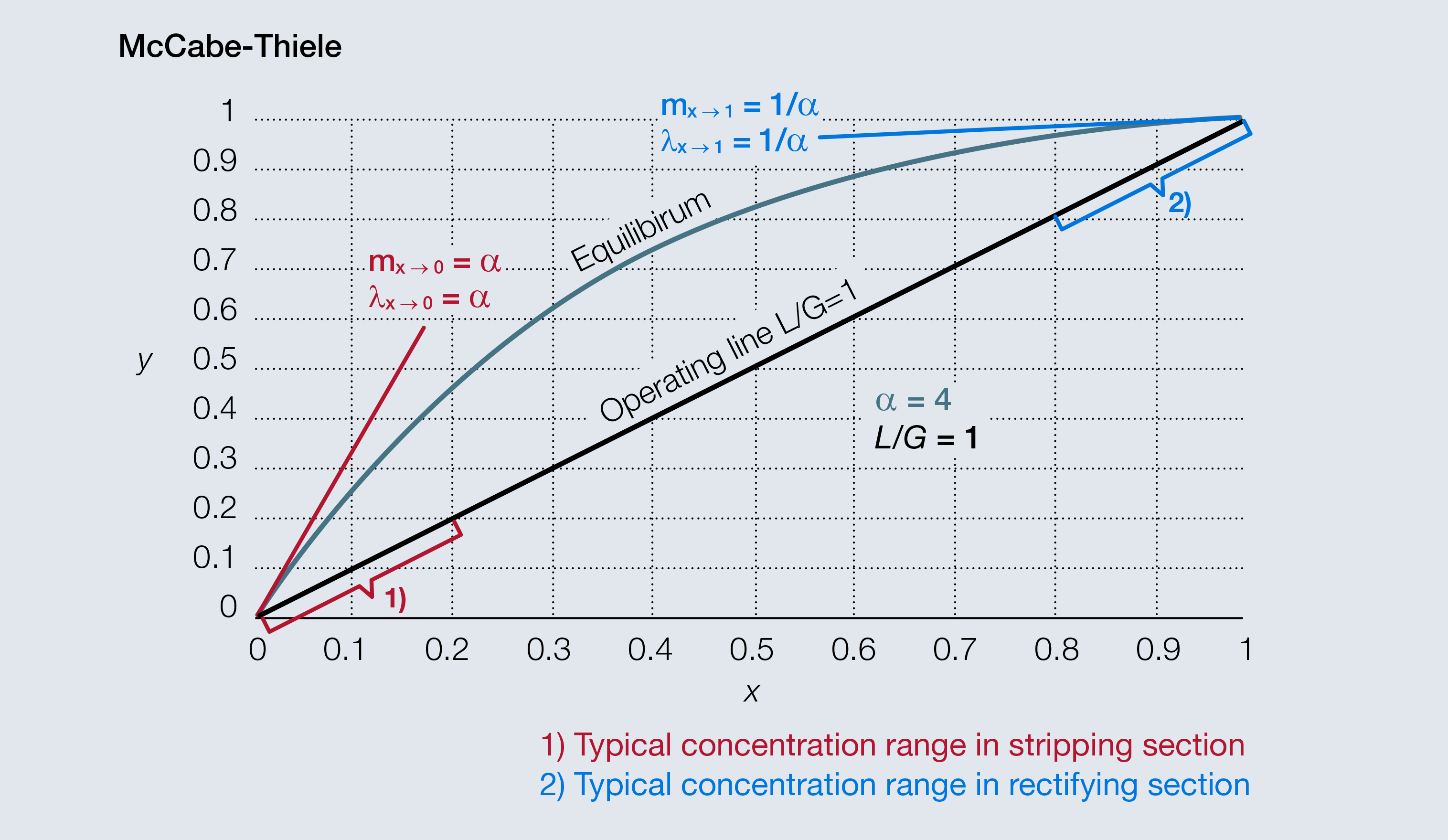Fig. 2 This McCabe-Thiele diagram for α = 4 shows the slope of the equilibrium line and the stripping factor at total reflux for x = 0 and x = 1.

Most distillation applications involve a relative volatility less than the α = 4 used in Fig. 2, and in such cases the difference between α and λ is less. For α < 1.3, no significant difference exists at any concentration.

#### 4. Revealing the ties

To establish the conditions under which the results of O’Connell’s correlation and the traditional model can agree, the stripping factor (λ), must be used instead of the relative volatility (α), and NG and NL must be expressed in terms of a single variable, namely the liquid viscosity. And, as in O’Connell’s approach, only one constant of proportionality and one fixed exponent will be used to correlate the number of transfer units. These simplifications avoid the cumbersome theoretical approach to calculate NG and NL. The correlations between NG and NL can be expressed with the same parameters in the form:

where c1 and x1 are constants determined by regression. Regressing c1 and x1 to minimize the difference between the outcome of the theoretical model (the left hand side of Eq. 8) and O’Connell’s correlation (the right hand side of Eq. 8) yields the proportionality constant c= 0.936 and the exponent x= – 0.25. Thus, Eq. 9 becomes:

Fig. 3 reveals the close agreement between the theoretical model and O’Connell’s empirical approach to estimating section efficiencies. The deviation over the range investigated is less than 10%. The section efficiencies obtained from the traditional model were calculated using the stripping factor for x = 0 and x = 1. Astonishingly, substituting Eq. 10 into Eq. 7 produces nearly identical results whether λ or 1/ λ is used. The reciprocal of the stripping factor often is referred to as the absorption factor.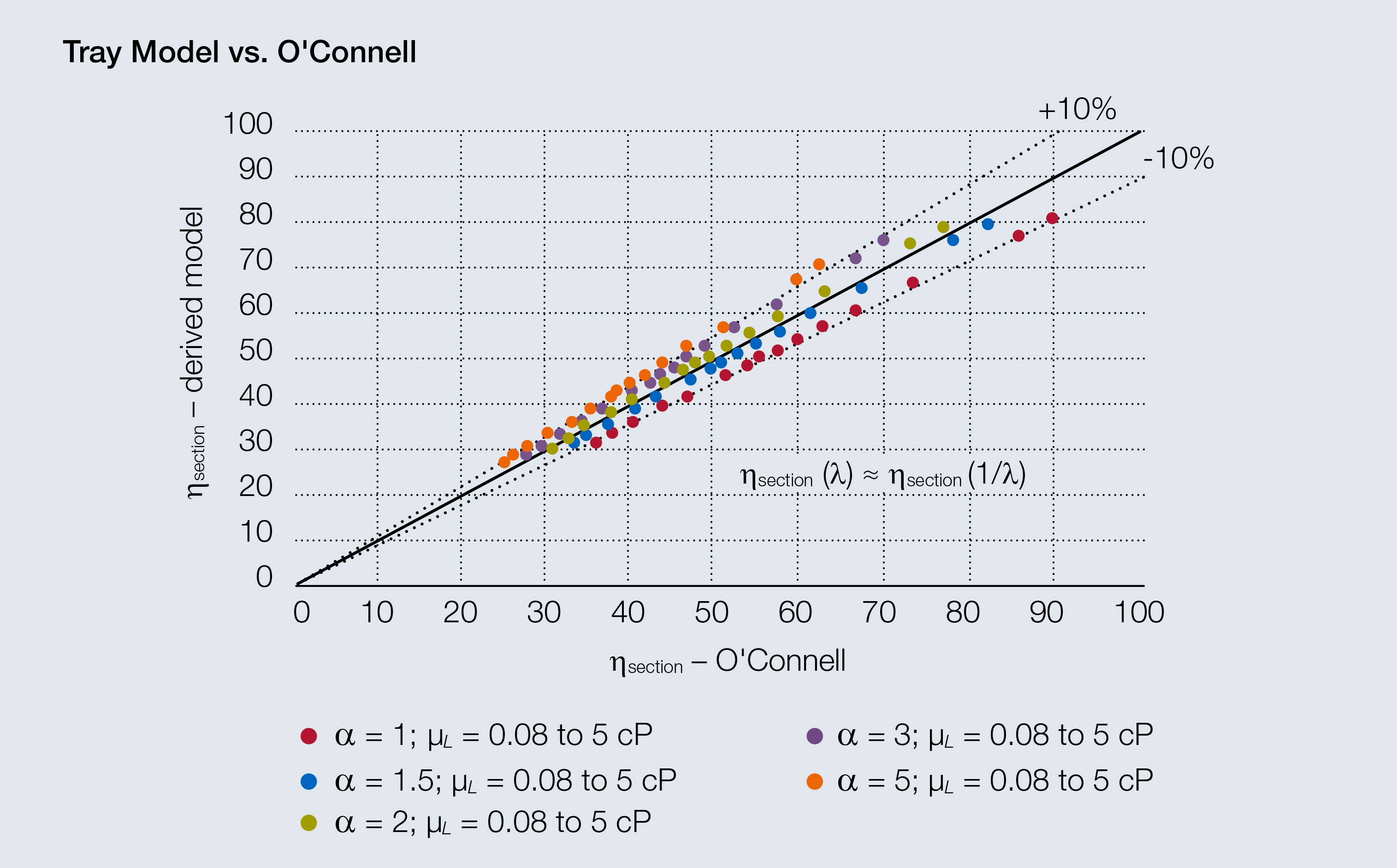Fig. 3 Parity plot of calculated section efficiencies for the O'Connell correlation and the derived model using Eqns. 7 and 10.

It is important to understand this outcome since this provides the justification for using the relative volatility instead of the stripping factor to represent relevant behavior. While the relative volatility is always greater than one, the stripping factor is smaller than one when the mixture contains high concentrations of the higher-boiling-point compound. Using the stripping factor based on α for x = 0 and x = 1 can, in many cases, reflect the approximate slope at total reflux in the striping and rectifying sections, respectively.

The dependency of the correlation on the liquid viscosity is very similar for the two correlations. However, the dependency on α or λ shows that O’Connell overestimates the impact of α on the section efficiency when compared to the model based on the traditional approach.

#### 5. Understanding the relationship

Combining Eq. 10 with Eq. 7 yields almost identical section efficiencies for a given value of the stripping factor and its reciprocal. Figure 4 plots the calculated section efficiencies for three different ratios of NG : NL . To allow a comparison, the stripping factor was varied and NG was adjusted in order to achieve the same maximum section efficiency to reveal apparent symmetries.

In the case of NG = NL (Fig. 4), there is an apparent symmetry about λ = 1. Such symmetry is not present in the other two cases. However, the NG = NL curve is not strictly symmetrical about λ = 1, as the efficiencies for values of λ and 1/ λ other than one are slightly different. The use of the relative volatility in O’Connell’s method has α theoretical basis only when such symmetry exists.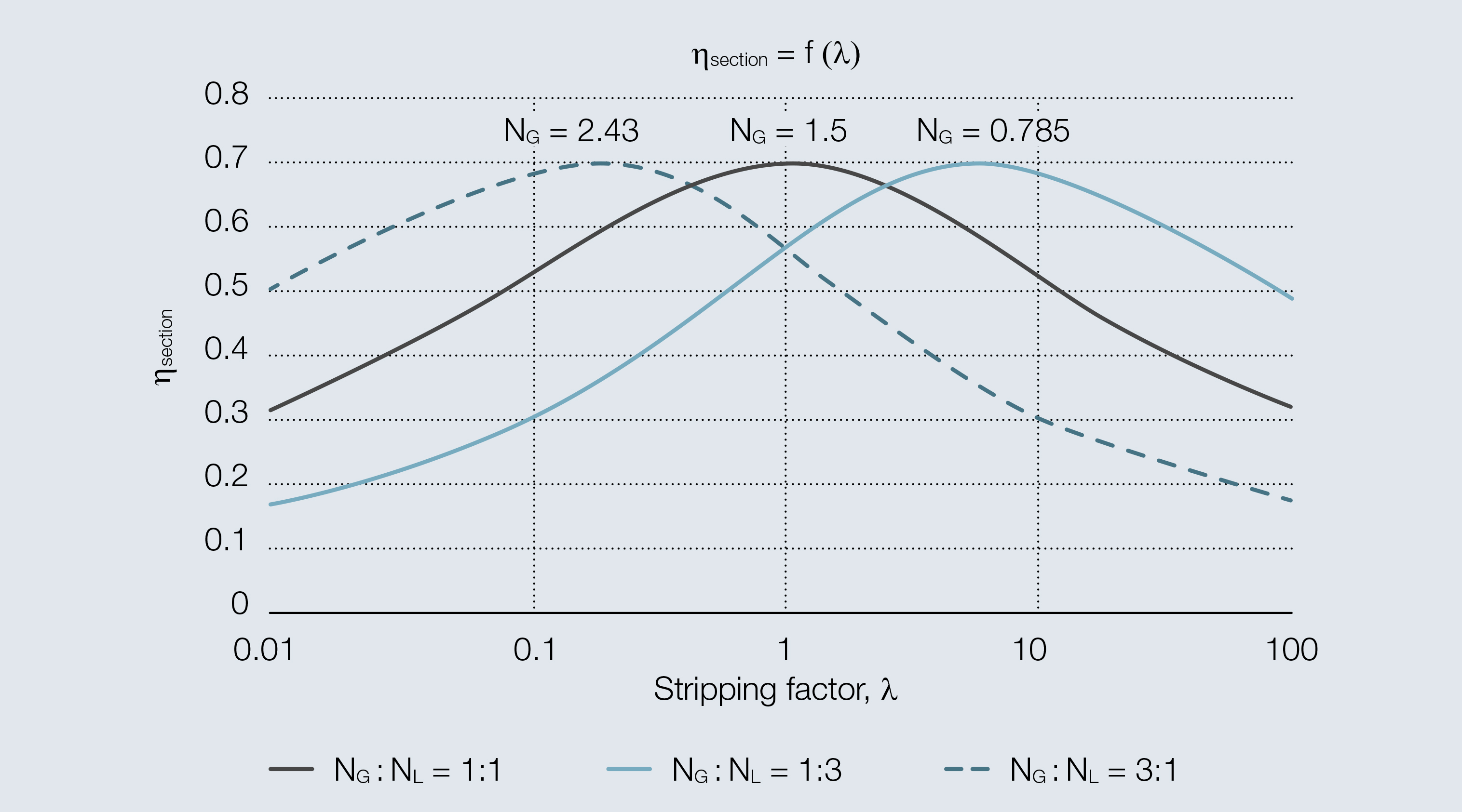Fig. 4 Calculated section efficiency depending on the stripping factor using Eqn. 7 for 3 different ratios of NG : NL, where the values are adjusted to achieve the same maximum efficiency.

The terms NG and NL quantify mass-transfer behavior in the vapor and liquid phases, respectively. Since the slope of the equilibrium line influences the distribution of the resistances to mass transfer across the two phases, assuming that NG = NL does not imply that the liquid-side and vapor-side resistances to mass transfer are equal. The fundamental relationship of the gas-side and liquid-side mass transfer coefficients is :

where KOG is the overall gas-phase mass-transfer coefficient in kmol/m2 x s and kG and kL are the gas-side and liquid-side mass-transfer coefficients, respectively, in kmol/m2 x s.

The relative liquid-phase resistance (LPR) in a column is defined as :

Fig. 5 shows the dependency of LPR on the stripping factor. The liquid-phase resistance increases with increasing stripping factor. For small values of λ, the liquid-phase resistance is negligible, whereas for high values of λ, LPR will become dominant, i.e., is more than 50% of the overall resistance.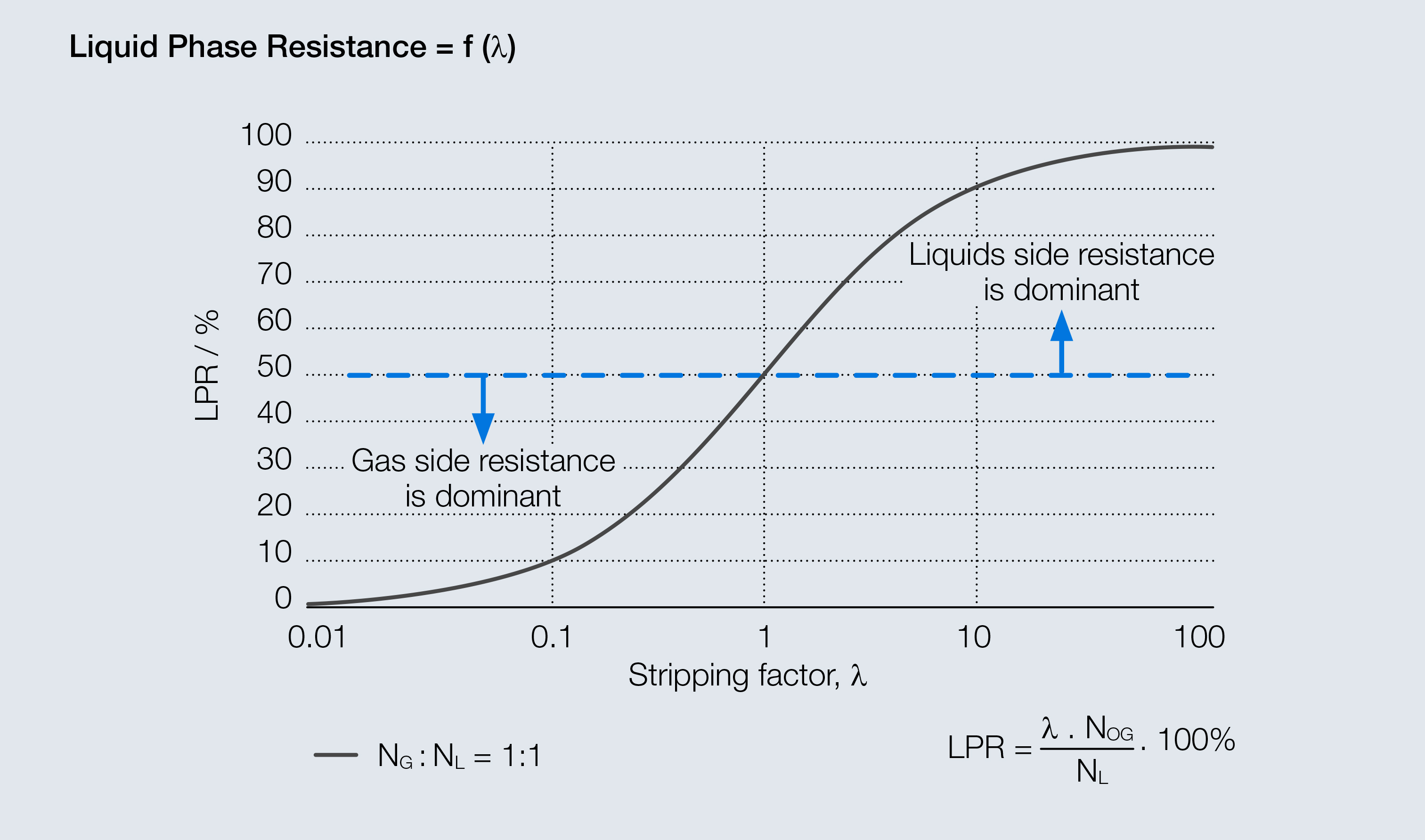Fig. 5 The liquid phase resistance depends on the stripping factor and the ratio of N G : N L, which is assumed to be unity for the shown example.
For increasing values of λ above one, the LPR increases and section efficiencies decrease. Thus, it would be reasonable to expect that with decreasing liquid resistance (i.e., for small values of λ), the section efficiency should increase, but this is not the case. To understand this behavior, it is important to quantify the impact of the stripping factor when converting the Murphree tray efficiency to the section efficiency. Fig. 6 illustrates the behavior of Eqs. 3 – 6, which are the basis for the derived model. The section efficiency calculated by Eq. 6 decreases with decreasing λ, explaining the drop in the section efficiency when the stripping factor becomes small, despite the fact that the Murphree tray efficiency increases. This explains why O’Connell’s correlation can use a instead of λ while still achieving meaningful results, provided the assumption NG = NL holds true.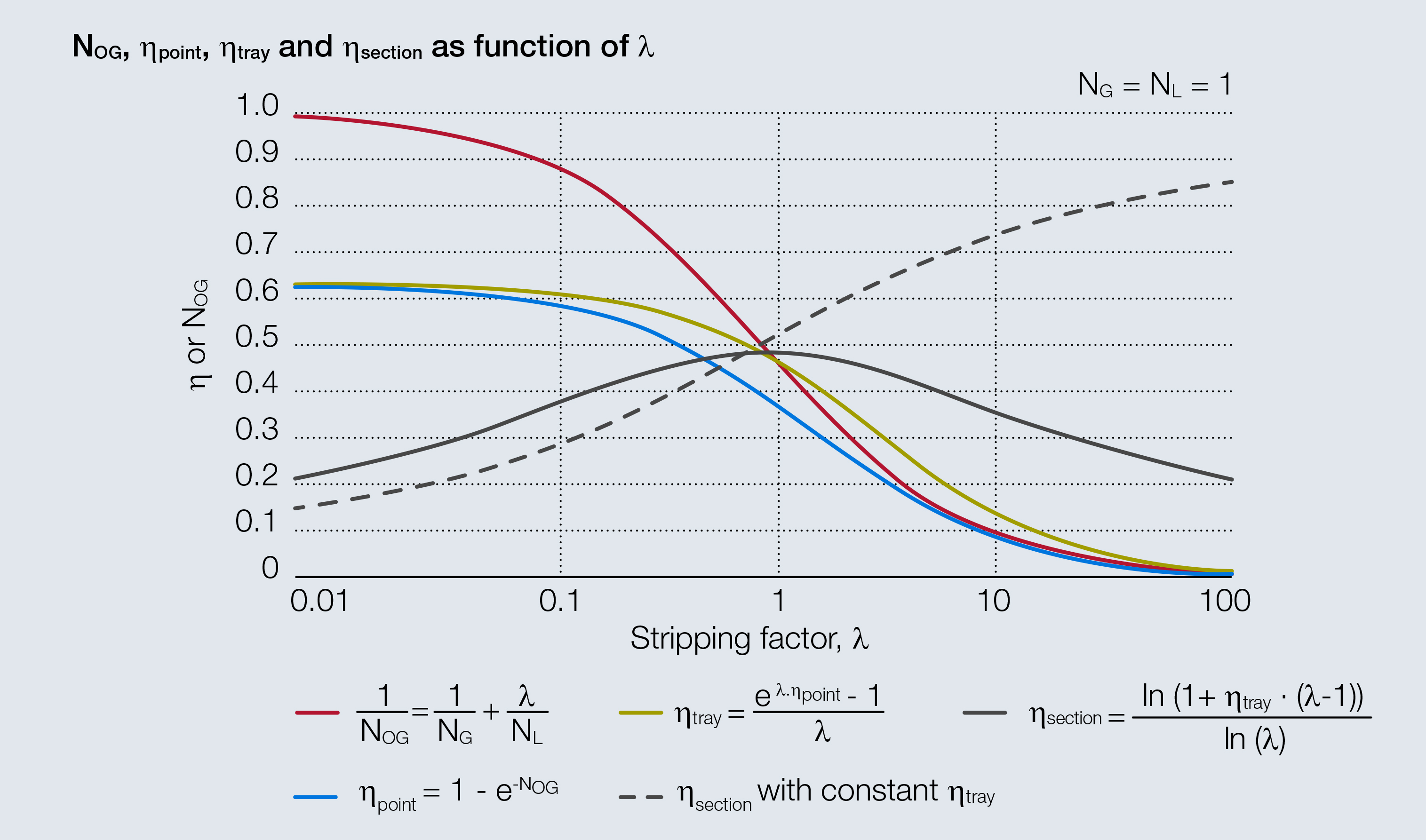Fig. 6 This graph shows the dependency of the relevant quantities to determine the section efficiency on the stripping factor assuming N G = N L = 1. The dotted line shows the section efficiency assuming a constant tray efficiency of 0.5.

In summary, for increasing values of λ, the liquid-phase resistance becomes dominant and reduces the section efficiency, whereas for decreasing values of λ, the driving force for mass transfer increases. The translation of the tray efficiency to section efficiency is the reason for the decrease of the section efficiency.

#### 6. Modifying the O’Connell correlation

The derived simplified theoretical model is expected to more accurately predict section efficiencies, albeit with increasing complexity. Therefore, a simple modification to O’Connell’s correlation better reflects theoretical outcomes.

The relative volatility affects the section efficiency in the O’Connell correlation more than it does in the theoretical model. Therefore, the exponent on α should be adjusted independently from the exponent on the liquid viscosity to better match the theoretical prediction.

Fig. 7 shows the relevant range for adjusting the exponent on α in the O’Connell correlation. The liquid viscosity was assumed to be 0.2 cP while α was varied between 1 and 5. The exponent on a was regressed and the best-match curve revealed the value to be – 0.08. The black line represents the theoretical dependency of the section efficiency on the stripping factor. The red line is the outcome using an exponent of – 0.08 on α for the modified O’Connell method.

The modified O’Connell correlation (Eq. 2) therefore becomes:Fig. 7 The modified O'Connell correlation can achieve a good agreement with the traditional model when the exponent for α is -0.08 if α < 5.
The modified O’Connell correlation (Eq. 2) therefore becomes:

The new exponent for a is now used to regress the proportionality constant (c1). Equation 10 is thus rewritten:

As the parity plot in Fig. 8 demonstrates, the modified O’Connell correlation (Eq. 13) achieves an almost-perfect fit with the combined Eqs. 7 and 14. Based on theoretical considerations, the stripping factor (λ) or its reciprocal (1/ λ) is preferred over the relative volatility (α). This is because when a is used, it is not possible to distinguish between total and partial reflux conditions; the stripping factor helps to account for this. Therefore, the modified O’Connell correlation can be written as:

where σ = λ if λ > 1 and σ = 1/λ if λ < 1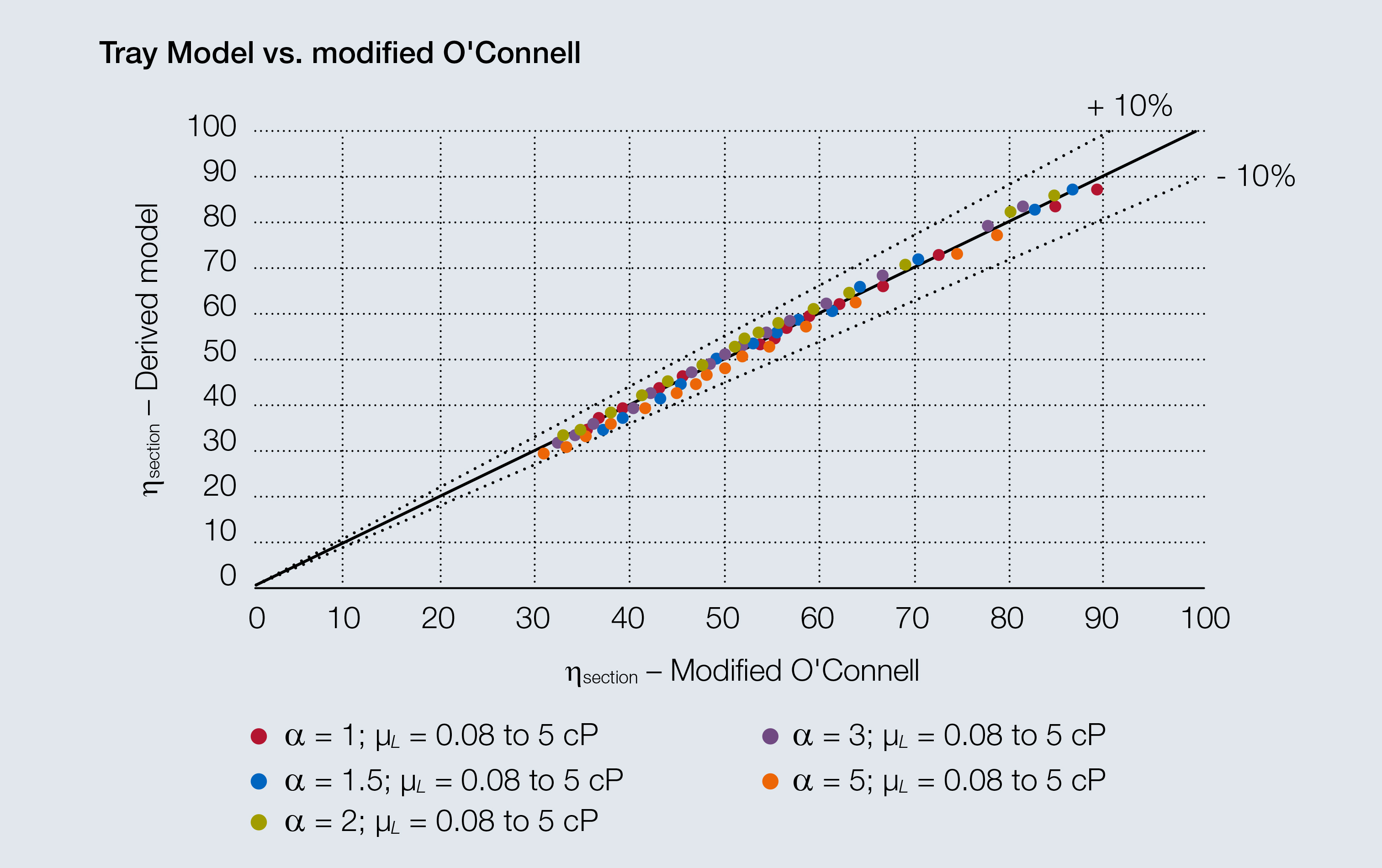Fig. 8 Parity plot of calculated section efficiencies for the modified O'Connell correlation and the derived model using Eqns. 7 and 14.

#### 7. Validation

When the O’Connell correlation was modified, the proportionality constant was retained at 0.503. Since this matched reasonably well with experimental data, the aim was not to alter the outcome for small values of α.

Chan evaluated more than 200 data points for six systems: cyclohexane/n-heptane, iso-butane/­n-butane, ethyl-benzene/styrene, water/acetic acid, methanol/water, and ammonia/water. He reported the section efficiency, the relative volatility, the average stripping factor, and the operating capacity . Chan’s data were used in order to compare the outcome of the original O’Connell correlation with the proposed modification. Data points for capacities of more than 98% or less than 25% were excluded from the analysis because the majority of those data points were associated with reduced efficiency due to jet flood or weeping, respectively.

Fig. 9 indicates that using the exponent of – 0.08 for the relative volatility improves the prediction of section efficiency. Using the stripping factor improves the accuracy further, but, more importantly, it allows Eq. 15 to be used for absorption and stripping applications as well as for distillation.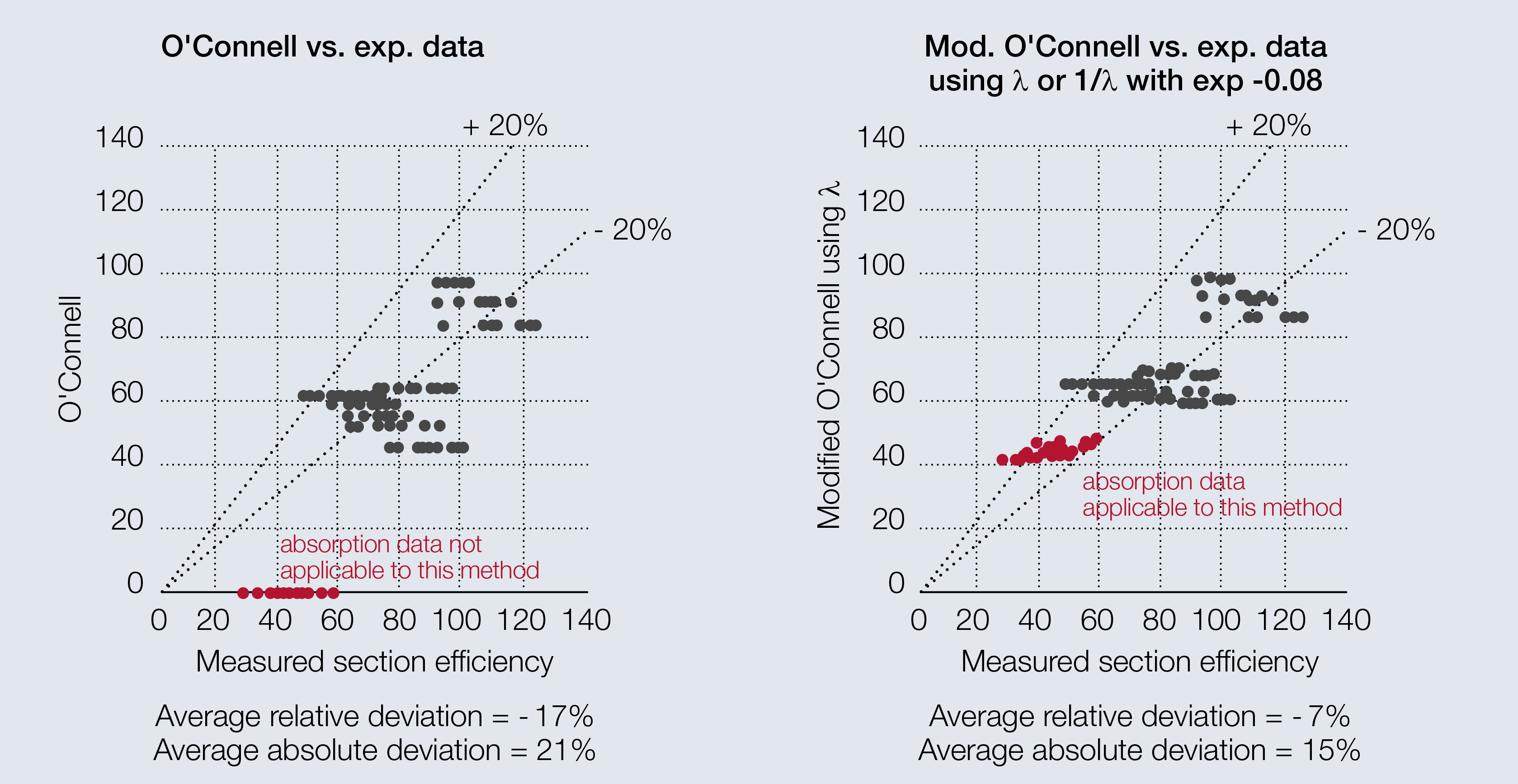Fig. 9 Parity plot of measured experimental section efficiencies and a) original O'Connell, b) modified O'Connell using λ.

#### 8. Closing summary

O’Connell’s empirical correlation is similar to the traditional theoretical tray efficiency model approach when the number of transfer units for the liquid phase and the vapor phase can be assumed to be equal. O’Connell’s use of the relative volatility approximates the stripping factor in the stripping section or its reciprocal in the rectifying section. Overall, the outcome of the O’Connell method agrees well with a theoretical model using the same number of parameters, namely a constant of proportionality, c1, and an exponent, x1, associated with the liquid viscosity.

The impact of liquid viscosity on the section efficiency should not be interpreted as representing only the liquid-side properties. Most distillation systems are gas-side controlled and are well represented by the approach shown here. Indeed, the liquid viscosity correlates well with other relevant properties, such as the effective interfacial area or the gas-phase residence time. A low liquid viscosity improves gas dispersion and can therefore increase the interfacial area.

Finding the reason for the success of O’Connell’s correlation and its connections to traditional theoretical efficiencies justifies its use in distillation applications while also revealing its limitations without the appropriate modifications.

#### 9. References

 O’Connell, H. E., “Plate Efficiency of Fractionating Columns and Absorbers,” Transactions of the American Institute of Chemical Engineers, 42, pp. 741–775 (1946).

 Lewis, W. K., “Rectification of Binary Mixtures,” Industrial and Engineering Chemistry, 28 (4), pp. 399–402 (1936).

 Lockett, M. J., “Distillation Tray Fundamentals,” Cambridge Univ. Press, New York, NY (1986).

 Chen, G. X., and K. T. Chuang, “Liquid-Phase Resistance to Mass Transfer on Distillation Trays,” Industrial and Engineering Chemistry Research, 34 (9), pp. 3078–3082 (1995).

 Chan, H., “Tray Efficiencies for Multicomponent Distillation Columns,” PhD Dissertation, the Univ. of Texas at Austin (May 1983).

##### Markus Duss

is a senior technical expert in mass transfer and hydraulics of structured and random packing at Sulzer Chemtech, Ltd. (Winterthur, Switzerland; Email: Markus.Duss@sulzer.com). He has worked with Sulzer Chemtech as a process engineer for more than 30 years and is a co-author of Distillation: Equipment and Processes (Elsevier, 2014). Duss graduated from the Zürich Univ. of Applied Sciences in Winterthur in 1983.

##### Ross Taylor

is the Kodak Distinguished Professor of Chemical Engineering at Clarkson University (Potsdam, NY; Email: rtaylor@clarkson.edu), where he teaches equipment and process design courses. He has held a joint appointment at the Univ. of Twente in The Netherlands and visiting appointments at Delft Univ. of Technology, BP Engineering in the U.K., and Shell Global Solutions in Amsterdam, Netherlands. Taylor is an author of Multicomponent Mass Transfer (Wiley, 1993) and of ChemSep, a software package for rate-­based column modeling. He is one of four authors of the distillation chapter in the 8th and 9th editions of Perry’s Chemical Engineers’ Handbook.

Sulzer Technical Review

Editor-in-Chief

Sulzer Management AG

Neuwiesenstrasse 15

8401 Winterthur

Switzerland